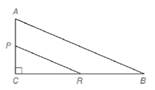Chapter 5.2, Problem 10EElementary Geometry For College St...

7th Edition
Alexander + 2 others
ISBN: 9781337614085

Solutions

Chapter
SectionElementary Geometry For College St...

7th Edition
Alexander + 2 others
ISBN: 9781337614085
Textbook Problem

Given: △ A B C ∼ △ P R C , m ∠ A = 67 ∘ , P C = 5 , C R = 12 , P R = 13 , A B = 26 Find: a) m ∠ B b) m ∠ R P C c) A C d) C BTo determine

a)

To calculate:

Find the angle mB.

Explanation

Given:

ΔABC~ΔPRCmA=67PC=5,CR=12,PR=13,and AB=26

Definition Used:

Sum of all the angles in a triangle is 180.

Calculate:

Here, mC=90

To determine

b)

To calculate:

Find the angle RPC.

To determine

c)

To calculate:

The value of AC.

To determine

d)

To calculate:

The value of CB.

Still sussing out bartleby?

Check out a sample textbook solution.

See a sample solution

The Solution to Your Study Problems

Bartleby provides explanations to thousands of textbook problems written by our experts, many with advanced degrees!

Get Started

Find the derivatives of the functions in Problems 1-34. 19.

Mathematical Applications for the Management, Life, and Social Sciences

In Exercises 5-8, graph the given function or equation. 2x3y=12

Finite Mathematics and Applied Calculus (MindTap Course List)

let f(x) = x3 + 5, g(x) = x2 2, and h(x)= 2x + 4. Find the rule for each function. 3. fg

Applied Calculus for the Managerial, Life, and Social Sciences: A Brief Approach

What is the value of (X + 1) for the following scores: 0, 1, 4, 2? a. 8 b. 9 c. 11 d. 16

Essentials of Statistics for The Behavioral Sciences (MindTap Course List)

an=5n

Calculus: Early Transcendental Functions (MindTap Course List)